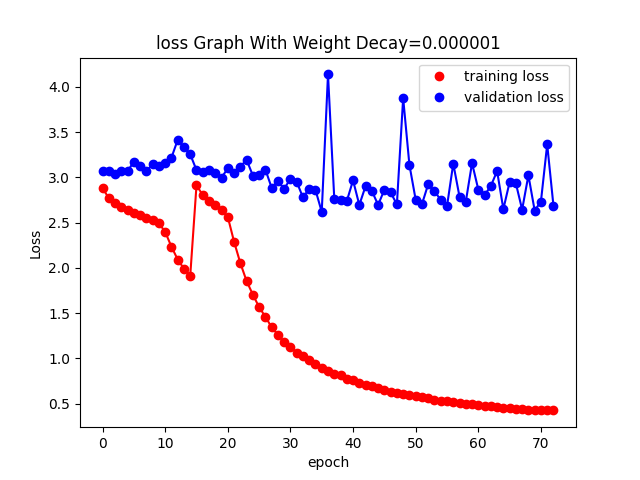# How to overcome this overfitting?

Hi,
I am trying to train a model for Handwritten text recognition task using encoder-decoder with attention. The loss of validtion is not decressing after reaching some value and train loss is getting decreseing and there is huge gap between them.
I have tried Dropout 0.5 after CNN fc layer and at the input of decoder. A Different weight decay from 0.1 to 0.000001 and also data augumenation have been applied.
I am using optim.Adam and nn.CrossEntropyLoss. My training exapmles are 8161 and validtion samples are 900.
Can anyone help me what is going wrong ?

Here is the loss:here is the Encoder:
class Encoder(nn.Module):
def init(self,height, width, enc_hid_dim, dec_hid_dim, dropout):
super().init()
self.height= height
self.enc_hid_dim=enc_hid_dim
self.width=width
self.num_layers=1

``````    self.layer0 = nn.Sequential(
nn.ReLU(),
nn.BatchNorm2d(8),
nn.MaxPool2d(2,2),
)
self.layer1 = nn.Sequential(
nn.ReLU(),
nn.BatchNorm2d(32),
nn.MaxPool2d(2,2),
)
self.layer2 = nn.Sequential(
nn.ReLU(),
nn.BatchNorm2d(64),
nn.MaxPool2d(2,2)
)
self.rnn=nn.LSTM(self.height//8*64, self.enc_hid_dim, num_layers= self.num_layers, bidirectional=True)

self.fc = nn.Linear(self.enc_hid_dim * 2, dec_hid_dim)

self.dropout_layer = nn.Dropout(dropout)

def forward(self, src, in_data_len, train):
batch_size = src.shape
out = self.layer0(src)
out = self.layer1(out)
out = self.layer2(out)
if train:
out = self.dropout_layer(out) # torch.Size([batch, channel, h, w])

out = out.permute(3, 0, 2, 1) # (width, batch, height, channels)
out.contiguous()
out = out.reshape(-1, batch_size, self.height//8*64) #(w,batch, (height, channels))
width = out.shape
src_len = in_data_len.numpy()*(width/self.width)
src_len = src_len + 0.999 # in case of 0 length value from float to int
src_len = src_len.astype('int')
outputs, hidden_out = self.rnn(out)

hidden=hidden_out
cell=hidden_out
# output: t, b, f*2  hidden: 2, b, f

#outputs = [src len, batch size, hid dim * num directions]
#hidden = [n layers * num directions, batch size, hid dim]
#hidden is stacked [forward_1, backward_1, forward_2, backward_2, ...]
#outputs are always from the last layer

#hidden [-2, :, : ] is the last of the forwards RNN
#hidden [-1, :, : ] is the last of the backwards RNN

hidden = torch.tanh(self.fc(torch.cat((hidden[-2,:,:], hidden[-1,:,:]), dim = 1)))
cell = torch.tanh(self.fc(torch.cat((cell[-2,:,:], cell[-1,:,:]), dim = 1)))

return outputs, hidden, cell``````

Awesome that you’ve implemented augmenting!

Main Suggestion: Given that the dataset is very small, reducing the overall complexity of your model should help to avoid overfitting. Perhaps try simply dropping one of the convolutional/sequential layers from your model and see what happens. (Note that to do this you’ll probably have to adjust the corresponding input layer of the LSTM.)

Side Note: Adjusting the Learning Rate α — and to a lesser extent, weight decay λ — in your call to optim.Adam() will help if you are having convergence issues, but it seems that your model is doing okay in that regard. (Commonly, λ ranges from `1e-5` to `1e-6`.) However, given the spike around epoch 15 it may still be worth looking into if the main suggestion doesn’t help.@AdamTogether Thanks for the reply. I am using only One layer BLSTM. First, I have tried 2 BLSTM layers in encoder, it was worse then 1 layer BLSTM.
So how we can be sure that the particualr problem is overfitting problem? As from the graph the validation loss is not increasing.
secondly, What does these spikes actaully represents? Why we are getting these spikes?

No problem - good that you checked different numbers of layers in BLSTM! If you have the choice of changing architectures, using transformers instead of BLSTM would likely perform much better in this case - though implementation can be a bit more difficult. However, I understand that sometimes we don’t have that choiceGenerally, if you are getting convergence on the training loss, but validation loss does not converge (in this case we see it increasing and decreasing rapidly), then your model is likely overfitting to the training dataset. (Assuming validation loss is being calculated correctly - which it is always good to double check lol.)

In terms of reducing model complexity, I would suggest you start by removing this layer:

``````    self.layer2 = nn.Sequential(
nn.ReLU(),
nn.BatchNorm2d(64),
nn.MaxPool2d(2,2)
)
``````

and maybe even removing this layer as well:

``````    self.layer1 = nn.Sequential(
In this case, I suspect the spike could be an indication that your Learning Rate α is a bit too large. (Learning rates that are too big will cause the gradient to explode during backpropagation, causing the training loss to oscillate.) In some cases however, seeing many small spikes in the training loss could simply be a result of optim.Adam using mini-batch. Perhaps try using different batch_sizes if reducing model complexity doesn’t help